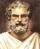Height 2

Calculate the height of the equilateral triangle with side 38.

Result

h =  32.9

Solution:Leave us a comment of example and its solution (i.e. if it is still somewhat unclear...):Be the first to comment!Next similar examples:

1. Broken treeThe tree is broken at 4 meters above the ground and the top of the tree touches the ground at a distance of 5 from the trunk. Calculate the original height of the tree.The ladder has a length 3.5 meters. He is leaning against the wall so that his bottom end is 2 meters away from the wall. Determine the height of the ladder.The double ladder shoulders should be 3 meters long. What height will the upper top of the ladder reach if the lower ends are 1.8 meters apart?
4. Chord 2Point A has distance 13 cm from the center of the circle with radius r = 5 cm. Calculate the length of the chord connecting the points T1 and T2 of contact of tangents led from point A to the circle.
5. An angleAn angle x is opposite side AB which is 10, and side AC is 15 which is hypotenuse side in triangle ABC. Calculate angle x.
6. Right triangleCalculate the length of the remaining two sides and the angles in the rectangular triangle ABC if a = 10 cm, angle alpha = 18°40'.
7. SAS triangleThe triangle has two sides long 7 and 19 and included angle 36°. Calculate area of this triangle.
8. Right triangle trigonometricsCalculate the size of the remaining sides and angles of a right triangle ABC if it is given: b = 10 cm; c = 20 cm; angle alpha = 60° and the angle beta = 30° (use the Pythagorean theorem and functions sine, cosine, tangent, cotangent)
9. SinesIn ▵ ABC, if sin(α)=0.5 and sin(β)=0.6 calculate sin(γ)
10. SemicircleIn the semicircle with center S and the diameter AB is constructed equilateral triangle SBC. What is the magnitude of the angle ∠SAC?
11. Cable carCable car rises at an angle 45° and connects the upper and lower station with an altitude difference of 744 m. How long is "endless" tow rope?
12. Cable car 2Cable car rises at an angle 41° and connects the upper and lower station with an altitude difference of 1175 m. How long is the track of cable car?
13. Triangle P2Can triangle have two right angles?
14. 30-60-90The longer leg of a 30°-60°-90° triangle measures 5. What is the length of the shorter leg?The double ladder is 8.5m long. It is built so that its lower ends are 3.5 meters apart. How high does the upper end of the ladder reach?What are the angles of an isosceles triangle ABC if its base is long a=5 m and has an arm b=4 m.It is true that the middle traverse bisects the triangle?